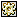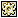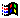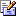Contents Articles Behaviors Books Director News Director Web Sites FAQ Games Mailing Lists News Groups Project Examples Reviews Software Tools Useful Web Sites Utilities Xtras

 Don't miss these#2D Collision from Explore Science

 Compatibilities:Rating:Author: RamanPfaff

This script is for the collision of two round objects (b <> 0). When your objects "collide" you call this script. Typically I would just check for distance between the two objects on an exitframe and when they "touch" (d ~ 0) I'd call this script, and with the results I would send a message to the objects and let them know what their velocities are after the collision.

 on dealWithCollision x1,y1,v1xi,v1yi,x2,y2,v2xi,v2yi,m1,m2   -- by Raman Pfaff (pfaff@ExploreScience.com)   -- This script is for the collision of two round objects (b <> 0).   -- when your objects "collide" you call this script. Typically I would   -- just check for distance between the two objects on an exitframe   -- and when they "touch" (d ~ 0) I"d call this script, and with the results   -- I would send a message to the objects and let them know what their   -- velocities are after the collision.      -- the initial parameters that you send to this script are as follows   -- the number (1 or 2) represent the two objects that have collided   -- x and y are the locations   -- the v"s are the velocity components (v1xi = initial x velocity of   -- object 1)   -- the m"s are the object masses      set term = pi()/180.  -- converting some radian stuff later      -- find the velocity magnitudes   set v1 = sqrt(power(v1xi,2)+power(v1yi,2))   set v2 = sqrt(power(v2xi,2)+power(v2yi,2))      -- find the direction of motion before the collision   set t1 = findThetaTan(v1xi,v1yi)   set t2 = findThetaTan(v2xi,v2yi)      -- find the angle of "contact" for the two objects   set phi = findThetaTan(x2-x1,y2-y1)         -- set coefficient of restitution here temporarily...set it elsewhere later.   -- this code set up for elastic...deal with inelastic differently...   set e = 1.      -- convert to a new reference frame for simplicity   -- now solve for relative velocities relative to "normal" reference frame   set v1xr = v1*cos((t1-phi)*term)   set v1yr = v1*sin((t1-phi)*term)   set v2xr = v2*cos((t2-phi)*term)   set v2yr = v2*sin((t2-phi)*term)      -- now find the final velocities in the normal reference frame   set v1fxr = ((m1-m2*e)*v1xr+(m2+m2*e)*v2xr)/(m1+m2)   set v2fxr = v1fxr +e*(v1xr-v2xr)   set v1fyr = v1yr   set v2fyr = v2yr      -- and convert those velocities back to the "standard" coordinate system   set v1fx = cos(phi*term)*v1fxr+cos((phi+90)*term)*v1fyr   set v1fy = sin(phi*term)*v1fxr+sin((phi+90)*term)*v1fyr   set v2fx = cos(phi*term)*v2fxr+cos((phi+90)*term)*v2fyr   set v2fy = sin(phi*term)*v2fxr+sin((phi+90)*term)*v2fyr      -- return the final velocities as a list   set temp = []   set temp = [v1fx,v2fy,v2fx,v2fy]   return temp end on findThetaTan xthing,ything   -- once again, this is hideous, but I kept copying it that week...I   -- should slap my other one in here...   -- one of these days.      set term = pi()/180.   if xthing < 0. then     set t = 180.+ atan(ything/xthing)/term   else if xthing > 0. and ything >= 0. then     set t = atan(ything/xthing)/term   else if xthing > 0. and ything < 0. then     set t = 360.+atan(ything/xthing)/term   else if xthing = 0. and ything = 0. then     set t=0.   else if xthing = 0. and ything >= 0. then     set t = 90.   else     set t = 270.   end if   return t end

 Contact MMI 36 South Court Sq Suite 300 Newnan, GA 30263 USASend e-mail